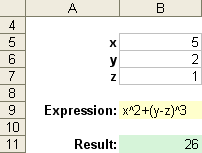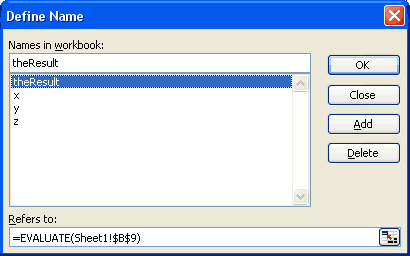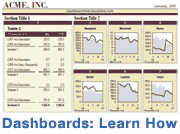I was asked once whether it was possible to get Excel to evaluate text as a formula, without defining a custom VBA function. For example, you may want to allow a person to enter an algebraic expression such as x^3+y as text (see the example below). When you want to create a formula using text from another cell, if the INDIRECT() function doesn't work for you and you can't get around the problem using nested IF statements or other tricks, you might want to try using the undocumented EVALUATE function.

I avoid using this function in my Excel Templates because of the reasons listed below and to avoid compatibility issues, but you can download a working example (see below).

I first came across the EVALUATE function when looking through Stephen Bullen's Excel Page. He has a great example of how you can graph an algebraic expression by just entering the expression as text in a cell.

The problem with the EVALUATE function (besides the fact that it is undocumented and may not work in all versions of Excel) is that it is actually a Microsoft Excel 4.0 Macro and when you open a file that uses this function, you will likely get a message asking if you want to enable macros (depending on your security settings).

Also, if you try to use this function within a cell, you'll get the error message "The function is not valid". The EVALUATE function can only be used in the definition of a Name. To define a Name, go to Insert > Name > Define in Excel 2003 or Formulas > Define Name in Excel 2007.

The benefit of the EVALUATE function is that you may be able to avoid having to define your own custom VBA function. This function works in Excel 2000, 2002(XP), 2003, and 2007. Because it is a macro, it doesn't work in Excel 2008 for the Mac.

### Example 1: Using the EVALUATE function

I'll first start off with a very simple example. Follow these steps:

1. Enter SUM(1+2) in cell A1 as text (don't put the "=" in front of SUM)
2. Define a Name called theResult (or choose your own name) and in the Refers to: field, enter =EVALUATE(A1)
3. To display the answer, enter =theResult in cell B1.

### Example 2: EVALUATE an Algebraic Expression

(You will need to enable the Excel 4.0 Macro)

The image below is a screenshot from the above file (evaluate-function.xls). This spreadsheet lets you enter values in cells B5 through B7 for x, y, and z respectively. Then, you can enter an expression in cell B9 and the result will be displayed in cell B11.To set up a spreadsheet like this, the expression in cell B9 is evaluated in the defined name "theResult" using the EVALUATE() function, as shown in the image below. The Named Ranges x, y, and z reference cells B5, B6, and B7, allowing you to use x in the expression instead of the cell reference B6.